# Random Number Generator – C PROGRAM

Wikipedia defines random numbers as

a sequence of numbers or symbols that cannot be reasonably predicted better than by a random chance

In this post I’m sharing various C programs and algorithms, that can be used to generate pseudo random numbers.
The reason these are called pseudo random numbers is because these are generated from computer algorithms, and hence not truly random and can be predicted if one knows the algorithm.

This might seem strange to you. How can an algorithm generate random numbers, how would it serve your purpose if you can predict the numbers etc. But once you read the whole post you will wrap your head around it.

So, one very simple and basic example of a random number generating algorithm would be to take a 4-digit number, square it and then take the middle 4-digits of the new squared number, and repeat the process. (NOTE: We won’t be using this one in our programs)

Here the starting 4-digit number that you take is called the ‘seed’. And the quality of your random number generator would depend on the starting number. For example 0000 would be a bad seed. Moreover, you would always get the same sequence of random numbers for the same seed, hence making the generation predictable.

This issue can be resolved by using some unpredictable seed. For example, if you’re making a game and want to make the moves of the enemy character unpredictable, then you could use the time difference between two events as a seed, and it would be impossible for the user to replicate the same time difference and hence making the moves truly random for the application.

Now, a popular algorithm (formula) to generate random numbers is:
1.$r_{i+1}=(a\times r_i)\mathrm{mod } m$
called the Linear Congruential Generator
This algorithm generates a maximum of$(m-1)$ random numbers with the maximum value of$m-1$ (Try to see why is it so).
Here,$r_i$ is the seed.
The values of$a$ and$m$ are carefully chosen values.

But what factors would you take into account while choosing these?

I’ll come back to it in a moment, but let’s just first write out a program and try a few values of$a$ and$m$ to generate some random numbers.

### CODE:

/********************************************
*********RANDOM NUMBER GENERATOR*************
*****PROGRAM TO GENERATE n RANDOM NUMBERS****
********************************************/
#include<stdio.h>
#include<math.h>
/**Function that generates a random number.
Parameters:
r0: initial (first) seed
a: scale factor , so that a*r0 give the first random number
m: gives the max. value of random numbers that can be generated (m-1)
**/
int rand(int r0, int a, int m){
int r1=(a*r0)%m;
return r1;
}
main(){
int a, m, r0, n;
printf("Enter the value of a:\n");
scanf("%d",&a);
printf("Enter the value of m:\n");
scanf("%d",&m);
printf("Enter the value of r0(initial):\n");
scanf("%d",&r0);
printf("Enter the no. of random nos. you require:\n");
scanf("%d",&n);
printf("The random nos. are:\n");
int i;
int r1=rand(r0,a,m);
for(i=0;i<n;i++){
printf("%d \n",r1);
r1=rand(r1,a,m);
}
}


### OUTPUT:

For$a=5$ and$m=37$ and$r0=1$ the output is:

You can see how the first random number that is generated will depend upon the seed value.
The numbers seem random enough. But is it really so? We’ll find out soon enough.

Now try for$a=1093$, and$m=86436$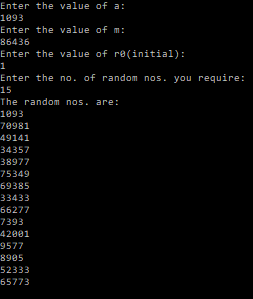Again, the numbers seem random at first look.

The following is a slight modification of the above program, where I’ve added a function that will store the random numbers in an array, other than that there is nothing new here.

### CODE:

/********************************************
*********RANDOM NUMBER GENERATOR2*************
********************************************/
#include<stdio.h>
#include<math.h>
/**Function that generates a random number.
Parameters:
r0: initial (first) seed
a: scale factor , so that a*r0 give the first random number
m: gives the max. value of random numbers that can be generated (m-1)
**/
int rand(int r0, int a, int m){
int r1=(a*r0)%m;
return r1;
}
/**Function that generates random numbers given a seed, and stores them in an array that is passed as an argument.
Parameters:
r0: initial (first) seed
a: scale factor , so that a*r0 give the first random number
m: gives the max. value of random numbers that can be generated (m-1)
n: no. of random numbers to be generated
x[n]: array that will store the random numbers
**/
void randomNos(int r0, int a, int m, int n, int x[n]){
int r1=rand(r0,a,m);;
int i;
for(i=0;i<n;i++){
x[i]=r1;
r1=rand(r1,a,m);
}
}
main(){
int a, m, r0, n;
printf("Enter the value of a:\n");
scanf("%d",&a);
printf("Enter the value of m:\n");
scanf("%d",&m);
printf("Enter the value of r0(initial):\n");
scanf("%d",&r0);
printf("Enter the no. of random nos. you require:\n");
scanf("%d",&n);
printf("The random nos. are:\n");
int i;
int randNos[n];
randomNos(r0, a, m, n, randNos);
for(i=0;i<n;i++){
printf("%d \n",randNos[i]);
}
}


### OUTPUT:

So I was talking about how do I check to see if the values of$a$ and$m$ that I am using are good for my usage or not.
Well, the first check would be to plot a distribution of random numbers. Let’s say your algorithm produces random numbers between 0 and 1. Then, ideally the number of random numbers generated in the windows$[0,0.1]$,$[0.1,0.2]$, etc. should be equal. Because there is no a priori reason for our algorithm to be preferring a particular number or range of numbers. Therefore, all numbers or ranges should be equally probable. Think of it this way. An unbiased coin, if tossed a lot of times, would give you almost the same number of Heads and Tails, thereby not preferring any particular outcome.

However, we will soon see that this test is not sufficient.

This brings me to another test, that is the correlation test.
For this, you could plot$r_{i+1}$ vs.$r_i$ and see if the graph shows any correlation.
Moreover, you could even repeat the process to see if there is any correlation between$r_{i+2}$ and$r_i$ ,$r_{i+3}$$r_i$, and so on.

It must be noted that the above checks and tests are not a sufficient to check our random number generator, as we will se in later posts. Therefore, it is often useful to try to model some real-life random process whose properties and behaviour are already known and well studied, and see if the random number generator is able to correctly reproduce that or not.

The following programs will illustrate the process.
In this program I will scale down the random numbers to lie between$[0,1]$ by dividing them by$(m-1)$ as that is the largest random number that can be generated. Then I will find out the frequency distribution within windows of width 0.1, and store these in a .txt file. Then I’ll also do a correlation test, where I will store$r_{i+1}$ and$r_i$ in a .txt file and then plot them to see any correlation.

### CODE:

/********************************************
*********RANDOM NUMBER GENERATOR*************
****POST-PROCESSING AND STATISTICAL CHECKS***
********************************************/
#include<stdio.h>
#include<math.h>
/**Function that generates a random number.
Parameters:
r0: initial (first) seed
a: scale factor , so that a*r0 give the first random number
m: gives the max. value of random numbers that can be generated (m-1)
**/
int rand(int r0, int a, int m){
int r1=(a*r0)%m;
return r1;
}
/**Function that generates random numbers given a seed, and stores them in an array that is passed as an argument.
Parameters:
r0: initial (first) seed
a: scale factor , so that a*r0 give the first random number
m: gives the max. value of random numbers that can be generated (m-1)
n: no. of random numbers to be generated
x[n]: array that will store the random numbers
**/
void randomNos(int r0, int a, int m, int n, int x[n]){
int r1=rand(r0,a,m);;
int i;
for(i=0;i<n;i++){
x[i]=r1;
r1=rand(r1,a,m);
}
}
main(){
int a, m, r0, n;
printf("Enter the value of a:\n");
scanf("%d",&a);
printf("Enter the value of m:\n");
scanf("%d",&m);
printf("Enter the value of r0(initial):\n");
scanf("%d",&r0);
printf("Enter the no. of random nos. you require:\n");
scanf("%d",&n);
int randNos[n];
randomNos(r0, a, m, n, randNos);
//Renormalize the randomnumbers so that their values are from within [0,1]
int i;
double randNosNew[n];
for(i=0;i<n;i++){
randNosNew[i]=(double)randNos[i]/(m-1);
}
//Begin distribution calculations within different intervals
int j;
double h=0.1; //width of interval
int count; //10 intervals of width 0.1
for(j=0;j<10;j++){
count[j]=0;
for(i=0;i<n;i++){
//find out the number of randomnumbers within an interval
if((j*h<=randNosNew[i])&&(randNosNew[i]<(j+1)*h)){
count[j]++;  //find out the number of randomnumbers within an interval
}
}
}
FILE *fp="NULL";
fp=fopen("randNosDistribution.txt","w");
for(i=0;i<10;i++){
fprintf(fp,"%lf\t%d\n",i*h,count[i]);
//printf("%d\n",count[i]);
}
//Correlation Checks
//Store r_{i} & r_{i+1} in a file and plot them to check for correlation
FILE *fp1="NULL";
fp1=fopen("randNosCorrelation.txt","w");
for(i=0;i<n-1;i++){
fprintf(fp1,"%d\t%d\n",randNos[i],randNos[i+1]);

}
}


### OUTPUT: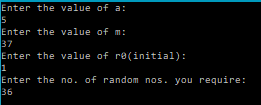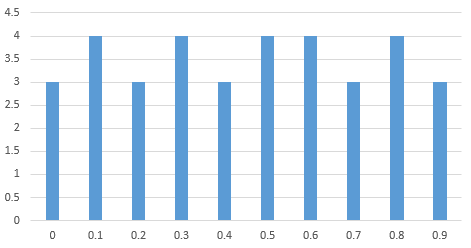Distribution of Random Numbers in windows of 0.1 after scaling down to [0,1]
0.000000 3 0.100000 4 0.200000 3 0.300000 4 0.400000 3 0.500000 4 0.600000 4 0.700000 3 0.800000 4 0.900000 3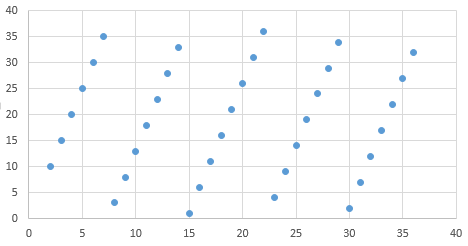Correlation check of r_{i+1} vs r_{i} for a=5 and m=37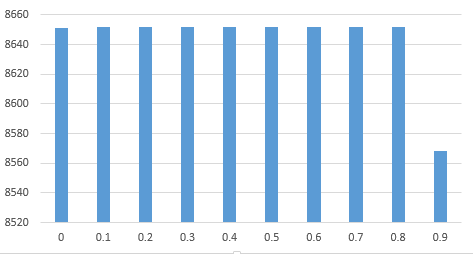Distribution of Random Numbers in windows of 0.1 after scaling down to [0,1]
0.000000 8651 0.100000 8652 0.200000 8652 0.300000 8652 0.400000 8652 0.500000 8652 0.600000 8652 0.700000 8652 0.800000 8652 0.900000 8568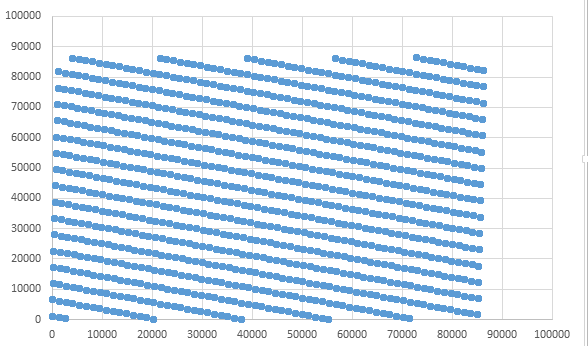Correlation check of r_{i+1} vs r_{i} for a=1093 and m=86436

So, we can see that both the pairs of values of a and m failed the correlation test and the distribution tests weren’t ideal either.

That is why mathematicians, spend a lot of time in choosing the correct set of values. Now, there is one set of value that is known to be pass the above tests, but I couldn’t verify it as the numbers were very large, and my program couldn’t handle these. The values are: a=16807 and m=2147483647 suggested by Par and Miller, who spent over 30 years surveying a large number of random number generators.

But now let me modify the above mentioned algorithm a little bit. Let’s add an offset parameter c.
So that the formula looks like:$r_{i+1}=(a\times r_{i} +c ) \mathrm{mod } m$

Now, let’s modify the above program to use this new formula and perform the above checks.

### CODE:

/********************************************
*********RANDOM NUMBER GENERATOR*************
***GENERATE RANDOM NUMBER USING (ari+c)mod m****
********************************************/
#include<stdio.h>
#include<math.h>
/**Function that generates a random number.
Parameters:
r0: initial (first) seed
a: scale factor , so that a*r0 give the first random number
m: gives the max. value of random numbers that can be generated (m-1)
c: additional displacement factor
**/
int rand(int r0, int a, int m, int c){
int r1=(a*r0+c)%m;
return r1;
}
/**Function that generates random numbers given a seed, and stores them in an array that is passed as an argument.
Parameters:
r0: initial (first) seed
a: scale factor , so that a*r0 give the first random number
m: gives the max. value of random numbers that can be generated (m-1)
c: additional displacement factor
n: no. of random numbers to be generated
x[n]: array that will store the random numbers
**/
void randomNos(int r0, int a, int m, int c, int n, int x[n]){
int r1=rand(r0,a,m,c);;
int i;
for(i=0;i<n;i++){
x[i]=r1;
r1=rand(r1,a,m,c);
}
}
main(){
int a, m, c, r0, n;
printf("Enter the value of a:\n");
scanf("%d",&a);
printf("Enter the value of m:\n");
scanf("%d",&m);
printf("Enter the value of c:\n");
scanf("%d",&c);
printf("Enter the value of r0(initial):\n");
scanf("%d",&r0);
printf("Enter the no. of random nos. you require:\n");
scanf("%d",&n);
int randNos[n];
randomNos(r0, a, m, c, n, randNos);

//Renormalize the randomnumbers so that their values are from within [0,1]
int i;
double randNosNew[n];
for(i=0;i<n;i++){
randNosNew[i]=(double)randNos[i]/(m-1);
}

//Begin distribution calculations within different intervals
int j;
double h=0.1; //width of interval
int count; //10 intervals of width 0.1
for(j=0;j<10;j++){
count[j]=0;
for(i=0;i<n;i++){
//find out the number of randomnumbers within an interval
if((j*h<=randNosNew[i])&&(randNosNew[i]<(j+1)*h)){
count[j]++;  //find out the number of randomnumbers within an interval
}
}
}

FILE *fp="NULL";
fp=fopen("randNosDistribution.txt","w");
for(i=0;i<10;i++){
fprintf(fp,"%lf\t%d\n",i*h,count[i]);
}
//Correlation Checks
//Store r_{i} & r_{i+1} in a file and plot them to check for correlation
FILE *fp1="NULL";
fp1=fopen("randNosCorrelation.txt","w");
for(i=0;i<n-1;i++){
fprintf(fp1,"%d\t%d\n",randNos[i],randNos[i+1]);

}
}


### OUTPUT:

Try the following values of a=1093, m=86436 and c=18257
and plot the distribution and correlation.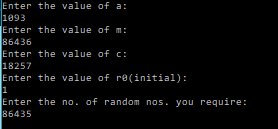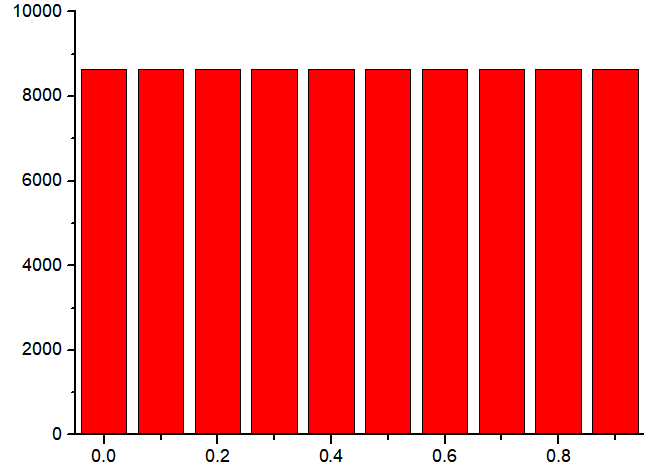Distribution of Random Numbers in windows of 0.1 after scaling down to [0,1]
0.000000 8643 0.100000 8643 0.200000 8644 0.300000 8643 0.400000 8644 0.500000 8644 0.600000 8643 0.700000 8643 0.800000 8644 0.900000 8643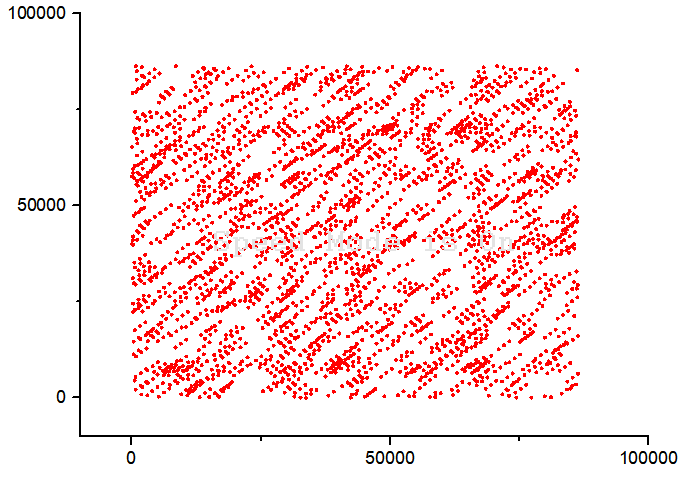Correlation check of r_{i+1} vs r_{i} for a=1093 and m=86436 and c=18257

Finally we see that the above set of values passes our checks, and hence would serve the purpose of use in our programs involving random number generation. (However, you will soon see some drawbacks in later posts.)

From now on, in future posts on random numbers applications, I will probably be using this new formula and the above set of values.

Now, that you have learnt a little something about random number generation, let’s talk about their applications.
Random numbers have wide range of applications, from something as simple as video games, gambling, etc to more advanced fields like computer simulations and cryptography.

They are very useful in Monte Carlo simulations. In cryptography they can be used to encrypt the data as long as the seed is kept a secret, which brings me to “True” and Cryptographically Secure Pseudo Random Number Generators (CSPRNG), which are random numbers that satisfy the criterion for use in the field of cryptography.

A “true” random number generator may rely on some natural phenomenon like radioactive decay, atmospheric noise, or some quantum phenomenon as to introduce randomness/entropy and hence generate something called a true random number.

This method may get a little too complicated or slow for practical use, and therefore the most popular algorithms are a hybrid of pseudo and true random number generators. They use natural sources to introduce randomness and fall back to periodically re-seeded software-based pseudo random number generators. The fallback occurs when the desired read rate of randomness exceeds the ability of the natural harvesting approach to keep up with the demand. This approach avoids the rate-limited blocking behavior of random number generators based on slower and purely environmental methods.

So if you’re looking for a new startup idea then you could look into the field of CSPRNGs.

### References and Resources:

https://en.wikipedia.org/wiki/Cryptographically_secure_pseudorandom_number_generator
Numerical Recipes in C[wpedon id="7041" align="center"]